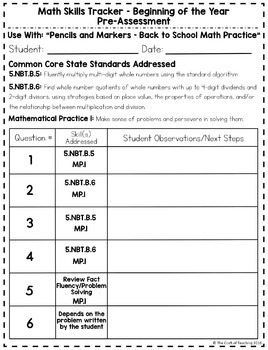Back to School Problem Solving 5th Grade5th
Subjects
Standards
Resource Type
Formats Included
• PDF
Pages
35 pages

Description

This 5th grade Problem Solving Pack has everything you need to gather baseline data about your new class of students! These word problems cover multiple 5th grade standards and are perfect for the beginning of the year.

You can observe your students' problem solving strategies, keep records of their progress and have a handy guide of a few great questions to ask students while they are working. This product includes detailed answer keys to help you evaluate your students' work toward mastering the standards.

The 5th Grade Problem Solving Pack focuses on the Number and Operations domain of the Common Core State Standards - operations with whole numbers and decimals and adding and subtracting fractions.

Included in this product:

• Step by step directions and ideas for using each assessment set
• 3 sets of word problems with detailed answer keys
• Individual Math Skills Tracker sheets for each set (organized by standard)
• Graphic organizers to help build and keep track of groups
• Individual Math Skill Tracker to use for any lesson
• Question Guide for questions to ask students (also in card format)

You may also like:

Math Talk Questions

Decimal Cards Activity Pack

Total Pages
35 pages
Included
Teaching Duration
N/A
Report this Resource to TpT
Reported resources will be reviewed by our team. Report this resource to let us know if this resource violates TpT’s content guidelines.

Standards

to see state-specific standards (only available in the US).
Solve word problems involving addition and subtraction of fractions referring to the same whole, including cases of unlike denominators, e.g., by using visual fraction models or equations to represent the problem. Use benchmark fractions and number sense of fractions to estimate mentally and assess the reasonableness of answers. For example, recognize an incorrect result 2/5 + 1/2 = 3/7, by observing that 3/7 < 1/2.
Add and subtract fractions with unlike denominators (including mixed numbers) by replacing given fractions with equivalent fractions in such a way as to produce an equivalent sum or difference of fractions with like denominators. For example, 2/3 + 5/4 = 8/12 + 15/12 = 23/12. (In general, 𝘢/𝘣 + 𝘤/𝘥 = (𝘢𝘥 + 𝘣𝘤)/𝘣𝘥.)
Add, subtract, multiply, and divide decimals to hundredths, using concrete models or drawings and strategies based on place value, properties of operations, and/or the relationship between addition and subtraction; relate the strategy to a written method and explain the reasoning used.
Find whole-number quotients of whole numbers with up to four-digit dividends and two-digit divisors, using strategies based on place value, the properties of operations, and/or the relationship between multiplication and division. Illustrate and explain the calculation by using equations, rectangular arrays, and/or area models.
Fluently multiply multi-digit whole numbers using the standard algorithm.